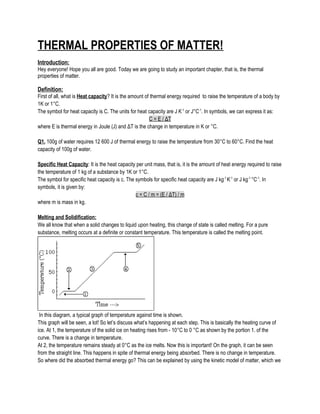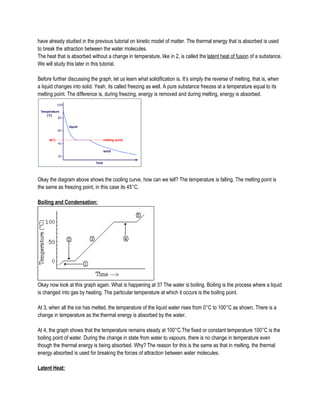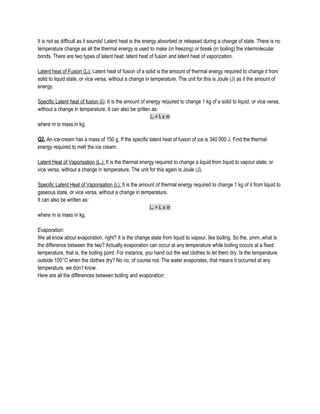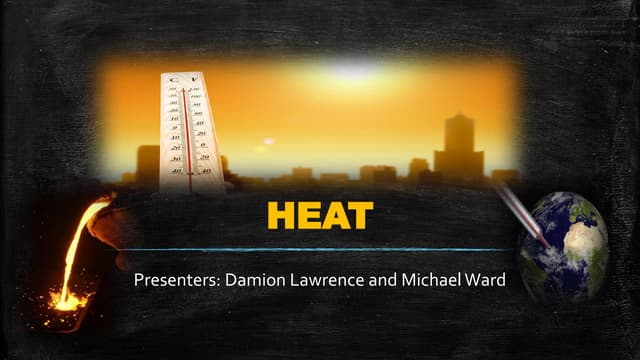PublicitéProchain SlideShareThermal energy edited
Chargement dans ... 3
1 sur 4
Publicité

### Thermal properties of matter

1. THERMAL PROPERTIES OF MATTER! Introduction: Hey everyone! Hope you all are good. Today we are going to study an important chapter, that is, the thermal properties of matter. Definition: First of all, what is Heat capacity? It is the amount of thermal energy required to raise the temperature of a body by 1K or 1°C. The symbol for heat capacity is C. The units for heat capacity are J K-1 or J°C-1 . In symbols, we can express it as: C = E / ΔT where E is thermal energy in Joule (J) and ΔT is the change in temperature in K or °C. Q1. 100g of water requires 12 600 J of thermal energy to raise the temperature from 30°C to 60°C. Find the heat capacity of 100g of water. Specific Heat Capacity: It is the heat capacity per unit mass, that is, it is the amount of heat energy required to raise the temperature of 1 kg of a substance by 1K or 1°C. The symbol for specific heat capacity is c. The symbols for specific heat capacity are J kg-1 K-1 or J kg-1 °C-1 . In symbols, it is given by: c = C / m = (E / ΔT) / m where m is mass in kg. Melting and Solidification: We all know that when a solid changes to liquid upon heating, this change of state is called melting. For a pure substance, melting occurs at a definite or constant temperature. This temperature is called the melting point. In this diagram, a typical graph of temperature against time is shown. This graph will be seen, a lot! So let’s discuss what’s happening at each step. This is basically the heating curve of ice. At 1, the temperature of the solid ice on heating rises from - 10°C to 0 °C as shown by the portion 1. of the curve. There is a change in temperature. At 2, the temperature remains steady at 0°C as the ice melts. Now this is important! On the graph, it can be seen from the straight line. This happens in spite of thermal energy being absorbed. There is no change in temperature. So where did the absorbed thermal energy go? This can be explained by using the kinetic model of matter, which we
2. have already studied in the previous tutorial on kinetic model of matter. The thermal energy that is absorbed is used to break the attraction between the water molecules. The heat that is absorbed without a change in temperature, like in 2, is called the latent heat of fusion of a substance. We will study this later in this tutorial. Before further discussing the graph, let us learn what solidification is. It’s simply the reverse of melting, that is, when a liquid changes into solid. Yeah, its called freezing as well. A pure substance freezes at a temperature equal to its melting point. The difference is, during freezing, energy is removed and during melting, energy is absorbed. Okay the diagram above shows the cooling curve, how can we tell? The temperature is falling. The melting point is the same as freezing point, in this case its 45°C. Boiling and Condensation: Okay now look at this graph again. What is happening at 3? The water is boiling. Boiling is the process where a liquid is changed into gas by heating. The particular temperature at which it occurs is the boiling point. At 3, when all the ice has melted, the temperature of the liquid water rises from 0°C to 100°C as shown. There is a change in temperature as the thermal energy is absorbed by the water. At 4, the graph shows that the temperature remains steady at 100°C.The fixed or constant temperature 100°C is the boiling point of water. During the change in state from water to vapours, there is no change in temperature even though the thermal energy is being absorbed. Why? The reason for this is the same as that in melting, the thermal energy absorbed is used for breaking the forces of attraction between water molecules. Latent Heat:
3. It is not as difficult as it sounds! Latent heat is the energy absorbed or released during a change of state. There is no temperature change as all the thermal energy is used to make (in freezing) or break (in boiling) the intermolecular bonds. There are two types of latent heat: latent heat of fusion and latent heat of vaporization. Latent heat of Fusion (Lf): Latent heat of fusion of a solid is the amount of thermal energy required to change it from solid to liquid state, or vice versa, without a change in temperature. The unit for this is Joule (J) as it the amount of energy. Specific Latent heat of fusion (lf): It is the amount of energy required to change 1 kg of a solid to liquid, or vice versa, without a change in temperature. It can also be qritten as: Lf = lf x m where m is mass in kg. Q2. An ice-cream has a mass of 150 g. If the specific latent heat of fusion of ice is 340 000 J. Find the thermal energy required to melt the ice cream. Latent Heat of Vaporisation (Lv): It is the thermal energy required to change a liquid from liquid to vapour state, or vice versa, without a change in temperature. The unit for this again is Joule (J). Specific Latent Heat of Vaporisation (lv): It is the amount of thermal energy required to change 1 kg of it from liquid to gaseous state, or vice versa, without a change in temperature. It can also be written as: Lv = lv x m where m is mass in kg. Evaporation: We all know about evaporation, right? It is the change state from liquid to vapour, like boiling. So the, umm..what is the difference between the two? Actually evaporation can occur at any temperature while boiling occurs at a fixed temperature, that is, the boiling point. For instance, you hand out the wet clothes to let them dry. Is the temperature outside 100°C when the clothes dry? No no, of course not. The water evaporates, that means it occurred at any temperature, we don’t know. Here are all the differences between boiling and evaporation:
4. Answers: Q1. 420 J K-1 Q2. 51 kJ
Publicité